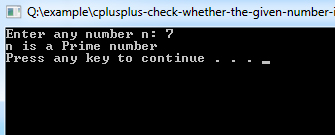## C++ Programming Examples Tutorial Index

A prime number is a natural number that has only one and itself as factors. This C++ program used to demonstrates how to find out whether a natural number is prime or not.

Examples: 2, 3, 13 are prime numbers.

Example:

``````#include <iostream>
using namespace std;

int main()
{
/* variable definition and initialization */
int n, i, c = 0;

/* Get user input */
cout << "Enter any number n: "; cin>>n;

/*logic*/
for (i = 1; i <= n; i++)
{
if (n % i == 0)
{
c++;
}
}
if (c == 2)
{
cout << "n is a Prime number" << endl;
}
else
{
cout << "n is not a Prime number" << endl;
}
return 0;
}``````

Program Output:Explanation:

First of all, you have to include the iostream header file using the "include" preceding by # which tells that hat the header file needs to be process before compilation, hence named preprocessor directive. Now, for removing naming conflict you can use namespace statement within a program.

Next, you have to declare three integer type variables 'n', 'i', 'c' and initialize c as 0. Now tell the user to enter any natural number n, using the cout<<""; statement. The 'cin<<' statement will take value from the input device, (here keyboard) and store it to the variable n. Now you have to implement a for - loop which will count from 1 up to n. And within this loop checks whether n divides with i gives value equals to 0 or not. If the condition becomes true increments the value of c.

Now when c == 2, prints that "n is a Prime number" and if c is having value other than 2, prints that "n is not a Prime number". And finally the return 0; statement is used to return an integer type value back to main().